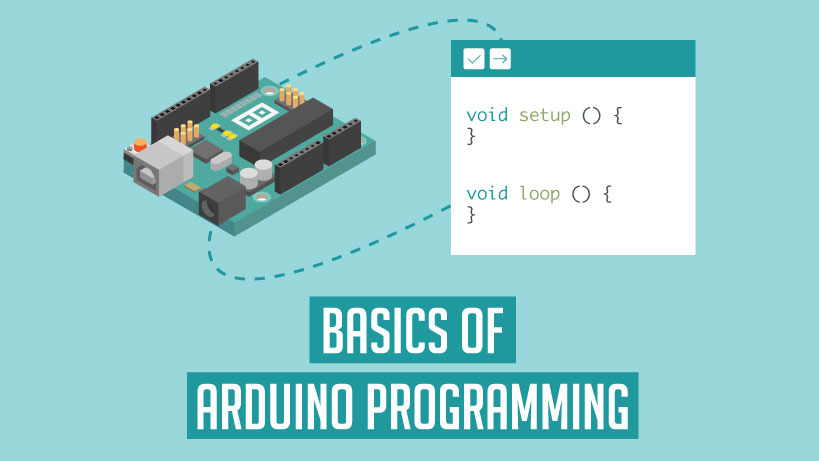# Arduino Programming Tutorial

Structure of Arduino Program

The basic structure of the Arduino programming language is fairly simple and runs in at least two parts. These two required parts, or functions, enclose blocks of statements.

void setup()

{

statements:

}

void loop()

{

statements;

}

Where, setup() is the preparation, loop() is the execution. Both functions are required for the program to work. The setup function should follow the declaration of any variables at the very beginning of the program. It is the first function to run in the program, is run only once, and is used to set pinMode or initialize serial communication. The loop function follows next and includes the code to be executed continuously-reading inputs, triggering outputs, etc. This function is the core of all Arduino programs and does the bulk of the work.

setup()

The setup() function is called once when your program starts. Use it to initialize pin modes, or begin serial. It must be included in a program even if there are no statements to run.

void setup()

{

pinMode(pin, OUTPUT);          //sets the ‘pin’ as output

}

loop()

After calling the setup() function, the loop() function does precisely what its name suggests, and loops consecutively, allowing the program to change, respond and control the Arduino board.

void loop()

{

digitalWrite(pin, HIGH);       //turns ‘pin’ on

delay(1000);                  //pauses for one second

digitalWrite(pin, LOW);      //turns ‘pin’ off

delay(1000);                  //pauses for one second

}

For Real-time Projects with Arduino refer to the video link:

Functions

A function is a block of code that has a name and a block of statements that are executed when the function is called. The functions void setup and void loop have already been discussed and other built-in functions will be discussed later.

Custom functions can be written to perform repetitive tasks and reduce clutter in a program. Functions are declared by first declaring the function type. This is the type of value to be returned by the function such as ‘int’ for an integer type function. If no value is to be returned the function type would be void. After type, declare the name given to the function and in parenthesis any parameters being passed to the function.

type functionName(parameters)

{

statements;

}

The following integer type function delayval() is used to set a delay value in a program by reading the value of a potentiometer. It first declares a local variable V, sets v to the value of the potentiometer which gives a number between 0-1023, then divides that value by 4 for a final value between 0-255, and finally returns that value back to the main program.

int delayval()

{

int v;                           //create temporary variable ‘v’

v/= 4;                           //converts 0-1023 to 0-255

return v;                        //return final value

}

{} Curly Braces

Curly braces (also referred to as just “braces” or “curly brackets”) define the beginning and end of function blocks and statement blocks such as the void loop() function and the for and if statements.

type function()

{

statements;

}

An opening curly brace { must always be followed by a closing curly brace) This is often referred to as the braces being balanced. Unbalanced braces can often lead to cryptic, impenetrable compiler errors that can sometimes be hard to track down in a large program.

The Arduino environment includes a convenient feature to check the balance of curly braces. Just select a brace, or even click the insertion point immediately following a brace, and its logical companion will be highlighted.

; semicolon

A semicolon must be used to end a statement and separate elements of the program. A semicolon is also used to separate elements in for a loop.

int x = 13;                    // declares variable ‘x’ as the integer 13

Note: Forgetting to end a line in a semicolon will result in a compiler error. The error text may be obvious, and refer to a missing semicolon, or it may not. If an impenetrable or seemingly illogical compiler error comes up, one of the first things to check is a missing semicolon, near the line where the compiler complained

Block comments or multi-line comments are areas of text ignored by the program and are used for large text descriptions of code or comments that help others understand parts of the program. They begin with / and end with / and can span multiple lines.

/*

this is an enclosed block comment don’t forget the closing comment they have to be balanced!

*/

Because comments are ignored by the program and take no memory space they should be used generously and can also be used to “comment out” blocks of code for debugging purposes.
Note: While it is possible to enclose single-line comments within a block comment, enclosing a second block comment is not allowed.

Single-line comments begin with // and end with the next line of code. Like block comments, they are ignored by the program and take no memory space.

// this is a single line comment

Single line comments are often used after a valid statement to provide more information about what the statement accomplishes or to provide a future reminder

Variables

A variable is a way of naming and storing a numerical value for later use by the program. As their namesake suggests, variables are numbers that can be continually changed as opposed to constants whose value never changes. A variable needs to be declared and optionally assigned to the value needing to be stored. The following code declares a variable called input variable and then assigns it the value obtained on analog input pin 2:

int inputVariable = 0;                    //declares a variable and

// assigns value of 0

inputVariable = analogRead(2);            //set variable to value of

// analog pin 2

inputVariable is the variable itself. The first line declares that it will contain an int, short for integer. The second line sets the variable to the value at analog pin2. This makes the value of pin 2 accessible elsewhere in the code.

Once a variable has been assigned, or re-assigned, you can test its value to see if it meets certain conditions, or you can use its value directly. As an example to illustrate three useful operations with variables, the following code tests whether the inputVariable is less than 100, if true it assigns the value 100 to inputVariable, and then sets a delay based on input variable which is now a minimum of 100:

if (inputVariable < 100)                      // tests variable if less than 100

{

input Variable = 100;          // if true assigns value of 100

}

delay (input Variable);        //uses variable as delay

Note: Variables should be given descriptive names, to make the code more readable. Variable names like tilt sensor or pushButton help the programmer and anyone else reading the code to understand what the variable represents. Variable names like var or value, on the other hand, do little to make the code readable and are only used here as examples. A variable can be named any word that is not already one of the keywords in the Arduino language

Variable Declaration

All variables have to be declared before they can be used. Declaring a variable means defining its value type, as in int, long, float, etc., setting a specified name, and optionally assigning an initial value. This only needs to be done once in a program but the value can be changed at any time using arithmetic and various assignments.

The following example declares that inputVariable is an int, or integer type and that its initial value equals zero. This is called a simple assignment.

int inputVariable = 0;

A variable can be declared in a number of locations throughout the program and where this definition takes place determines what parts of the program can use the variable.

Variable Scope

A variable can be declared at the beginning of the program before void setup(). locally inside of functions, and sometimes within a statement block such as for loops. Where the variable is declared determines the variable scope, or the ability of certain parts of a program to make use of the variable.

A global variable is one that can be seen and used by every function and statement in a program. This variable is declared at the beginning of the program, before the setup() function.

A local variable is one that is defined inside a function or as part of a for a loop. It is only visible and can only be used inside the function in which it was declared. It is therefore possible to have two or more variables of the same name in different parts of the same program that contain different values. Ensuring that only one function has access to its variables simplifies the program and reduces the potential for programming errors.

The following example shows how to declare a few different types of variables and demonstrates each variable’s visibility:

int value;                //’value’ is visible to any function

void setup()

{

// no setup needed

}

void loop()

{

for (int i=0; i<20;)    //’i’ is only visible

{                           //inside the for-loop

i++;

}

float f;                     //’f’ is only visible

}                            //inside loop

byte

Byte stores an 8-bit numerical value without decimal points. They have a range of 0-255

Byte  someVariable = 180;                 // declares some Variable as a byte type

int

Integers are the primary datatype for the storage of numbers without decimal points and store a 16bit value with a range of 32,767 to -32,768.

int someVariable = 1500;                  //declares some Variable as an integer type

Note: Integer variables will roll over if forced past their maximum or minimum values by an assignment or comparison. For example, if x = 32767 and a subsequent statement adds 1 to x, X= X + 1 or x++, X will then rollover and equal -32,768.

long

Extended size datatype for long integers, without decimal points, stored in a 32-bit value with a range of 2.147.483,647 to -2,147,483,648.

long someVariable = 90000;                //declares ‘some Variable’ as a long type

float

A data type for floating-point numbers, or numbers that have a decimal point. Floating-point numbers have greater resolution than integers and are stored as a 32-bit value with a range of 3.4028235E+38 to -3.4028235E+38.

float someVariable = 3.14;                //declares ‘someVariable’ as a floating-point type

Note: Floating-point numbers are not exact, and may yield strange results when compared. Floating-point math is also much slower than integer math in performing calculations, so should be avoided if possible.

arrays

An array is a collection of values that are accessed with an index number. Any value in the array may be called upon by calling the name of the array and the index number of the value. Arrays are zero-indexed, with the first value in the array beginning at index number 0. An array needs to be declared and optionally assigned values before they can be used.

Likewise, it is possible to declare an array by declaring the array type and size and later assign values to an index position:

int myArray;         //declares integer array w/ 6 positions

myArray = 10;        //assigns the 4th index the value 10

To retrieve a value from an array, assign a variable to the array and index position:

x = myArray;         //x now equals 10

Arrays are often used for loops, where the increment counter is also used as the index position for each array value. The following example uses an array to flicker an LED. Using a for loop, the counter begins at 0, writes the value contained at index position 0 in the array flicker, in this case, 180, to the PWM pin 10, pauses for 200ms, then moves to the next index position.

int ledPin = 10;                                               // LED on pin 10

byte flicker[] = {180, 30, 255, 200, 10, 90, 150, 60};

// above array of 8 different values

void setup()

{

pinMode(ledPin, OUTPUT);                          // sets OUTPUT pin

}

void loop()

{

for(int i=0; i<7; i++)                                        // loop equals number of values in array

{

analogWrite(ledPin, flicker[i]);                        // write index value

delay(200);                                                      // pause 200ms

}

}

arithmetic

Arithmetic operators include addition, subtraction, multiplication, and division.They return the sum, difference, product, or quotient (respectively) of two operands.

y = y + 3;

x = x – 7;

i = j * 6;

r = r / 5;

The operation is conducted using the data type of the operands, so, for example, 9/4 results in 2 instead of 2.25 since 9 and 4 are ints and are incapable of using decimal points. This also means that the operation can overflow if the result is larger than what can be stored in the data type.

If the operands are of different types, the larger type is used for the calculator For example, if one of the numbers (operands) are of the type float and the other of type integer, floating-point math will be used for the calculation.

Choose variable sizes that are large enough to hold the largest results from your calculations.

Know at what point your variable will roll over and also what happens in the other direction e.g. (0 – 1) OR (0 — 32768). For math that requires fractions, use float variables, but be aware of their drawbacks: large size and slow computation speeds.

Note: Use the cast operator e.g. (int)myFloat to convert one variable type to another on the fly. For example, i = (int)3.6 will set i equal to 3.

Compound Assignments

Compound assignments combine an arithmetic operation with a variable assignment. These are commonly found in for loops as described later. The most common compound assignments include:/p>
x  ++                                        // same as x = x + 1, or increments x by +1

x  —                                          // same as x = x – l, or decrements x by – 1

x +=y                                       // same as x = x + y, or increments x by+y

x -=y                                        // same as x = x – y, or decrements x by-y

x *=y                                       // same as x = x * y, or multiplies x by y

x /= y                                       // same as x = x / y, or divides x by y

Note: For example, x= 3 would triple the old value of x and re-assign the resulting value to x.

Comparison Operators

Comparisons of one variable or constant against another are often used in if statements to test if a specified condition is true. In the examples found on the following pages, ?? is used to indicate any of the following conditions:

x == y                          // x is equal to y

x != y                           // x is not equal to y

х< у                             // x is less than y

x > y                            // x is greater than y

x <= y                          // x is less than or equal to y

x >= y                          // x is greater than or equal to y

Logical Operators

Logical operators are usually a way to compare two expressions and return a TRUE or FALSE depending on the operator. There are three logical operators, AND, OR, and NOT, that are often used in if statements:

Logical AND:

if (x > 0 && x < 5)                  // true only if both

// expressions are true

Logical OR:

if (x > || y > 0)                         // true if either

// expression is true

Logical NOT:

if (!x > 0)                                 // true only if

//expression is false

Constants

The Arduino language has a few predefined values, which are called constants. They are used to make the programs easier to read. Constants are classified into groups.

True/False

These are Boolean constants that define logic levels. FALSE is easily defined as 0 (zero) while TRUE is often defined as 1, but can also be anything else except zero. So in a Boolean sense, -1, 2, and -200 are all also defined as TRUE

if (b == TRUE);

{

doSomething;

}

High/Low

These constants define pin levels as HIGH or LOW and are used when reading or writing to digital pins. HIGH is defined as logic level 1, ON, or 5 volts while LOW is logic level O, OFF, or 0 volts.

digitalWrite(13, HIGH);

input/output

Constants are used with the pinMode() function to define the model of a digital pin as either INPUT or OUTPUT.

pinMode(13, OUTPUT);

if

if statements test whether a certain condition has been reached, such as an analog value being above a certain number, and executes any statements inside the brackets if the statement is true. If false the program skips over the statement. The format for an if the test is:

if (someVariable ?? value)

{

doSomething;

}

The above example compares someVariable to another value, which can be either a variable or constant. If the comparison, or condition in parentheses is true, the statements inside the brackets are run. If not, the program skips over them and continues on after the brackets.

Note: Beware of accidentally using ‘=’, as in if(x-10), while technically valid, defines the variable x to the value of 10 and is as a result always true. Instead use ‘ ‘, as in if(x==10), which only tests whether x happens to equal the value 10 or not. Think of ‘=’ as “equals opposed to ‘=’ being “is equal to”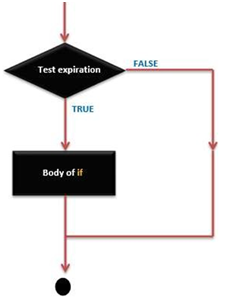if…else

if…else allows for ‘either-or’ decisions to be made. For example, if you wanted to test a digital input, and do one thing if the input went HIGH, or instead do another thing if the input was LOW. you would write that this way

if (inputPin == HIGH)

{

do ThingA;

}

else

{

do ThingB;

}

else can also precede another test, so that multiple, mutually exclusive tests can be run at the same time. It is even possible to have an unlimited number of these else branches. Remember though, only one set of statements will be run depending on the condition tests:

if (inputPin < 500)

{

do ThingA;

}

else if (inputPin >= 1000)

{

do ThingB;

}

else

{

do ThingC;

}

Note: An if statement simply tests whether the condition inside the parenthesis is true or false. This statement can be any valid C statement as in the first example, if (inputPin == HIGH). In this example, the if statement only checks to see if indeed the specified input is at a logic level high, or +5v.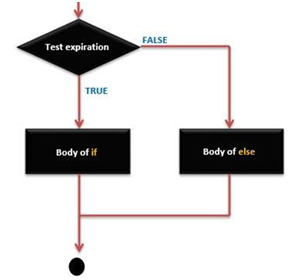for

The for statement is used to repeat a block of statements enclosed in curly braces a specified number of times. An increment counter is often used to increment and terminate the loop. There are three parts, separated by semicolons (:), to the for loop header:

for (initialization; condition; expression)

{

doSomething;

}

The initialization of a local variable, or increment counter, happens first and only once. Each time through the loop, the following condition is tested. If the condition remains true, the following statements and expressions are executed and the condition is tested again. When the condition becomes false, the loop ends. The following example starts the integer i at 0, tests to see if i is still less than 20 and if true, increments i by 1 and executes the enclosed statements: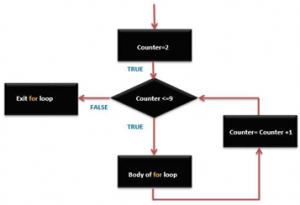for (int i=0; i<20; i++)                        // declares i, tests if less

{                                                                      // than 20, increments i by 1

digitalWrite(13, HIGH);                                 // turns pin 13 on

delay(250);                                                      // pauses for 1/4 second

digitalWrite(13, LOW);                                  // turns pin 13 off

delay(250);                                                      // pauses for 1/4 second

}

Note: The C for loop is much more flexible than for loops found in some other computer languages, including BASIC. Any or all of the three header elements may be omitted, although the semicolons are required. Also, the statements for initialization, condition, and expression can be any valid C statements with unrelated variables. These types of unusual statements may provide solutions to some rare programming problems.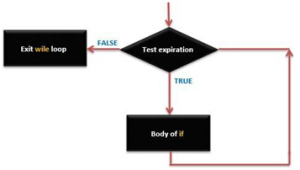While

while loops will loop continuously, and infinitely until the expression inside the parenthesis becomes false. Something must change the tested variable, or the while loop will never exit. This could be in your code, such as an incremented variable, or an external condition, such as testing a sensor.

while (someVariable ?? value)

{

doSomething;

}

The following example tests whether ‘someVariable is less than 200 and if true executes the statements inside the brackets and will continue looping until ‘someVariable is no longer less than 200.

While (someVariable < 200) // tests if less than 200

{

doSomething;                                                  // executes enclosed statements

someVariable++;                                             // increments variable by 1

}

do… while

The do loop is a bottom-driven loop that works in the same manner as the while loop, with the exception that the condition is tested at the end of the loop, so the do loop will always run at least once.

do

{

doSomething;

} while (someVariable ?? value);

The following example assigns readSensors() to the variable ‘x’. pauses for 50 milliseconds, then loops indefinitely until ‘x’ is no longer less than 100:

do

{

delay(50);                                            // pauses 50 milliseconds

} while (x < 100);                              // loops if x is less than 100

Close
Viewed

Close